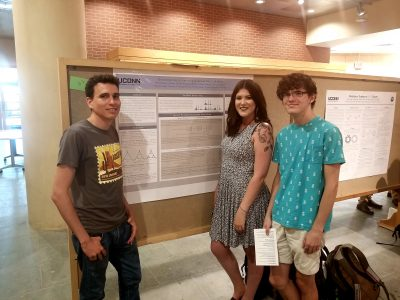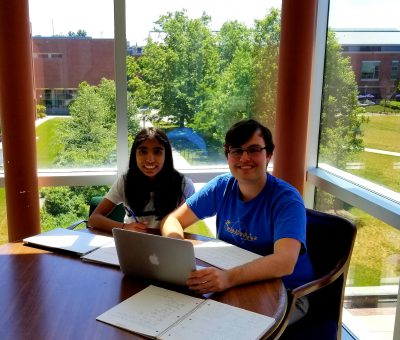# Decimation structure of the spectra of self-similar groups

## Group Members## Overview

Physicists and mathematicians have used the self-similar nature of certain fractals to develop and study analytical structures on fractal spaces. We examine the analytical structure of a class of fractals that arise as limit sets of the Schreier graphs of the action of self-similar groups on infinite n-ary trees. In particular, we consider how the spectrum of a Laplacian operator on one level of a Schreier graph relates to the spectrum on the next level, a technique known as spectral decimation.

Grigorchuk and collaborators have developed a method to spectrally decimate Schreier graphs of several
important self-similar groups, and have derived significant consequences about the structure of amenable groups. Their method is related to a notion of spectral similarity arising from the work of Fukushima-Shima and Malozemov-Teplyaev. In the latter, a sufficient condition for spectral decimation for fractal graphs is obtained. We consider the analogous question for Schreier graphs of self-similar groups with the goal of understanding the class to which Grigorchuk’s approach is applicable.

# Hedging by Sequential Regression in Generalized Discrete Models and the Follmer-Schweizer decomposition## Overview

In practice, financial models are not exact — as in any field, modeling based on real data introduces some degree of error. However, we must consider the effect error has on the calculations and assumptions we make on the model.  In complete markets, optimal hedging strategies can be found for derivative securities; for example, the recursive hedging formula introduced in Steven Shreve’s “Stochastic Calculus for Finance I” gives an exact expression in the binomial asset model, and as a result the unique arbitrage-free price can be computed at any time for any derivative security.

In incomplete markets this cannot be accomplished; one possibility for computing optimal hedging strategies is the method of sequential regression.  We considered this in discrete-time; in the (complete) binomial model we showed that the strategy of sequential regression introduced by Follmer and Schweizer  is equivalent to Shreve’s recursive hedging formula, and in the (incomplete) trinomial model we both explicitly computed the optimal hedging strategy predicted by the Follmer-Schweizer decomposition and we showed that the strategy is stable under small perturbations.

# Can we hear the shape of a fractal? Spectral analysis of self-similar sets

## Group Members## Overview

Analytic structures on fractals have been analyzed extensively in the past 50 years both because of their interesting mathematical properties and their potential applications in physics. One important question in this area is how the spectrum of a Laplacian on a fractal reflects its geometry; one version of the corresponding problem for domains in Euclidean space was famously described in Kac’s question ”Can you hear the shape of a drum?”.
Some features of the spectra of self-similar sets, such as the asymptotic behavior of the eigenvalue counting function, can be obtained using renewal theory (as in the work of Kigami-Lapidus), but our interest is in more precise results that give the locations and multiplicities of eigenvalues explicitly. These are connected to a long strand of research in mathematical physics about the structure of spectra of Schrodinger operators ¨ and their relation to topological invariants of the underlying space (prominent results in this area are due to Landau, Peierls, Harper, Moser, Bellissard, and, recently, Avila and Jitomirskaya). One name for these results is gap-labeling theorems. For certain highly-symmetric self-similar sets, the computation of the gap structure of the Laplacian spectrum is possible using spectral decimation. We use this method to explicitly compute the gap structure for the Laplacian on a particular two-point self-similar graph and its fractal limit, and for Sierpinski graphs and the Sierpinski gasket.

# Resistance scaling on the Octacarpet

## Group Members## Overview

The study of analytic structures on self-similar fractal sets was initiated by physicists who discovered that heat flow on such sets had sub-Gaussian rather than Gaussian scaling, indicating that the fundamental physics of these sets was very different than on manifolds. These results were first made rigorous for sets with a finite ramification property, but in the late 1980s, Barlow and Bass developed a corresponding theory on a class of generalized Sierpinski carpets. Their approach depends on taking a (weak) limit of Brownian motions on a suitable sequence of closed sets that intersect to the carpet. A key step in proving that the limiting object has sub-Gaussian scaling is showing that the resistance of the approximating domain of scale n is bounded above and below by ρ^n
for a factor ρ that depends on the carpet. Computing the exact value of ρ remains an open problem.

We consider the resistance scaling problem for the octacarpet, and more generally for 4N-carpets, with
the goal of showing analogous bounds for the resistance and obtaining numerical estimates for the resistance scaling factors.

# REU Sigma Seminar 2019

Seminars convene every Thursday during the REU program at 12:30 pm in MONT 214.

August 1st at 10:30 am: Keith Conrad, The Biggest Known Prime Number.

Abstract: Since there are infinitely many prime numbers, there is no biggest prime. But there is always a biggest known prime, currently over 24 million digits. It belongs to a family of primes called Mersenne primes. The talk will discuss the history of Mersenne primes and how their primality is checked.

July 25: Gianmarco Molino, Heat Kernels and Index Theory.

Abstract: TBA.

July 18: Matthew Badge, Curves, and Parameterizations.

Abstract: I will introduce some ideas in metric geometry. A curve is the image of a continuous map from [0,1] into a metric space. How do we measure the length of a curve? How do we tell whether or not a set of points is a curve of finite length, and if it is, how do we build a map whose image is the curve? If time permits, I will also describe analogues of these questions for higher-dimensional curves, which are open for research.

July 11: Behrang Forghani, Harmonic Functions, and Trees.

Abstract: In this talk, I will introduce the notion of bounded harmonic functions and discuss some of their properties for Markov chains.
D. Blackwell classified the space of bounded harmonic functions for random walks on Z. I will present his elegant proof and other results related to regular trees.

July 4: No Seminar.

June 27: Arthur Parzygnat, Computing the square root of a positive matrix.

Abstract: Given any function f on some domain and a diagonalizable nxn matrix A whose eigenvalues are in the domain of f, we will prove there exists a polynomial p such that p(A)=Pf(D)P^{-1}, where P is a matrix of eigenvectors of A and D is the corresponding matrix of eigenvalues. This may sound strange when you look at examples. For instance, given a (positive) matrix A, we will find a polynomial p such that p(A)=sqrt(A), the square-root of A. Along the way of proving this theorem, we will learn several useful techniques and results. For example, we will prove that if B is a matrix that commutes with A, i.e. AB=BA, then f(A)B=Bf(A) for any function f (satisfying the above assumptions).

June 20: Reed Solomon, Graduate School Panel.

Abstract: Want to learn about graduate school in mathematics and the application process. Ask Professor Reed Solomon (Director of Graduate Studies) and Ph.D. students Waseet Kazmi, Gianmarco Molino, Sean Eustace, and Lisa Naples!

June 13: Christopher Hayes, Introduction to self-similar structures.

Abstract: One category of a fractal is the self-similar set. Famous examples include the Sierpinski Carpet and Koch Curve and analysis of these types of fractals is currently an active field of research. In this talk we will go over a variety of examples, discuss some construction methods, definitions, commonly used properties such as the open set condition and having a finite post-critical set, and end with comments on how analysis can be done on these sets, in particular, the construction of a Dirichlet form on a post-critically finite set.

# UCONN Math REU at REU Conference at UMass Amherst 2019

Our REU participants will be giving talks on July 23rd, 2019 at the REU Conference at UMass Amherst 2019.

Fractals projects:

• Resistance scaling on the Octacarpet  (Claire Canner, William Huang, Michael Orwin)
• Decimation structure of the spectra of self-similar groups (Brett Hungar, Madison Phelps, Johnathan Wheeler)
• Can we hear the shape of a fractal? Spectral analysis of self-similar sets (Elizabeth Melville, Nikhil Nagabandi)

Stochastic and Financial Mathematics project:

• Hedging by Sequential Regression in Generalized Discrete Models and the Follmer-Schweizer
decomposition (Sarah Boese, Tracy Cui, Sam Johnston)

Markov Chains projects:

• A Version of the Elephant Random Walk and Additive Functionals of Finite State Markov Chains (Jonah Green, Taylor Meredith, Rachel Tan)
• The Voter Model on Complete Bipartite Graphs ( Philip Speegle, R Oliver Vandenberg)
• On a Nonlinear Random Walk on Graphs (Jonah Botvinick-Greenhouse, Connor Fitch, Mark Kong)

Additional information and abstract list: REU Conference at UMass Amherst 2019

# Geodesic Interpolation on the Sierpinski Gasket

## Overview

Geodesics (shortest paths) on manifolds such as planes and spheres are well understood.  Geodesics on fractal sets such as the Sierpinski Triangle are much more complicated.  We begin by constructing algorithms for building shortest paths and provide explicit formulas for computing their lengths.  We then turn to the question of interpolation along geodesics—given two subsets of the Sierpinski Triangle, we “slide” points in one set along geodesics to the other set.  We construct a measure along the interpolated sets which formalizes a notion of the interpolation of a distribution of mass, and we prove interesting self-similarity relations about this measure.

# Computations on the Koch Snowflake with Boundary and Interior Energies

## Group Members## Overview

At the end of the 20th century studies had been conducted on the Koch Snowflake which had been motivated through work done by physicists on “fractal drum” experiments. These investigations focused on the eigenfunctions of the negative Dirichlet Lapcian generated on a planar domain with a fractal boundary, particularly with the condition that the boundary be set to zero. Here we study the eigenfunctions on the Koch Snowflake with a non-zero boundary condition and we consider a Laplacian defined on the boundary. To generate an n-level fractal and apply the Laplacian matrix, Python programming was implemented for both the n-level fractal and Laplacian matrix. This then gives us insight into the eigenvalues and visualization of the corresponding eigenfunctions on the Koch Snowflake. Initial observations indicate a kind of localization of the eigenfunctions on the fractal boundary.

# Lipschitz Continuity of Laplacian Eigenfunctions on a class of postcritically finite (PCF) self-similar sets

## Group Members## Overview

We prove a general result for Lipschitz and Hölder continuity of functions defined on a class of postcritically finite (PCF) self-similar sets. Intuition for this theorem comes from formulating arguments on the unit interval, which is well understood in a classical setting. We generalize these results for many kinds of self-similar sets endowed with different measures and metrics. As a corollary to this general result, we prove that eigenfunctions of the Laplacian on the harmonic Sierpinski Gasket are Lipschitz continuous.